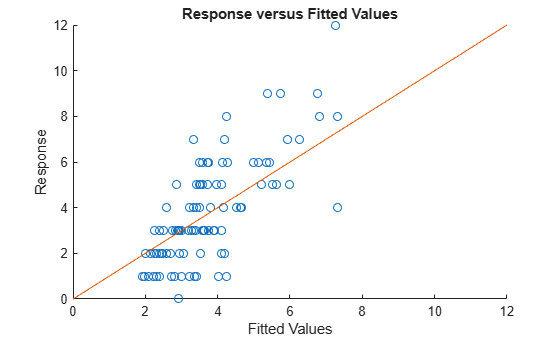# response

Response vector of generalized linear mixed-effects model

## Syntax

``y = response(glme)``
``````[y,binomialsize] = response(glme)``````

## Description

example

````y = response(glme)` returns the response vector `y` used to fit the generalized linear mixed effects model `glme`.```
``````[y,binomialsize] = response(glme)``` also returns the binomial size associated with each element of `y` if the conditional distribution of response given the random effects is binomial.```

## Input Arguments

expand all

Generalized linear mixed-effects model, specified as a `GeneralizedLinearMixedModel` object. For properties and methods of this object, see `GeneralizedLinearMixedModel`.

## Output Arguments

expand all

Response values, specified as an n-by-1 vector, where n is the number of observations.

For an observation i with prior weights wip and binomial size ni (when applicable), the response values yi can have the following values.

DistributionPermitted ValuesNotes
`Binomial`

`$\left\{0,\frac{1}{{w}_{i}^{p}{n}_{i}},\frac{2}{{w}_{i}^{p}{n}_{i}},\dots ,1\right\}$`

wip and ni are integer values > 0
`Poisson`

`$\left\{0,\frac{1}{{w}_{i}^{p}},\frac{2}{{w}_{i}^{p}},\dots \right\}$`

wip is an integer value > 0
`Gamma`(0,∞)wip ≥ 0
`InverseGaussian`(0,∞)wip ≥ 0
`normal`(-∞,∞)wip ≥ 0

You can access the prior weights property wip using dot notation. For example, to access the prior weights property for a model `glme`:

`glme.ObservationInfo.Weights`

Binomial size associated with each element of `y`, returned as an n-by-1 vector, where n is the number of observations. `response` only returns `binomialsize` if the conditional distribution of response given the random effects is binomial. `binomialsize` is empty for other distributions.

## Examples

expand all

`load mfr`

This simulated data is from a manufacturing company that operates 50 factories across the world, with each factory running a batch process to create a finished product. The company wants to decrease the number of defects in each batch, so it developed a new manufacturing process. To test the effectiveness of the new process, the company selected 20 of its factories at random to participate in an experiment: Ten factories implemented the new process, while the other ten continued to run the old process. In each of the 20 factories, the company ran five batches (for a total of 100 batches) and recorded the following data:

• Flag to indicate whether the batch used the new process (`newprocess`)

• Processing time for each batch, in hours (`time`)

• Temperature of the batch, in degrees Celsius (`temp`)

• Categorical variable indicating the supplier (`A`, `B`, or `C`) of the chemical used in the batch (`supplier`)

• Number of defects in the batch (`defects`)

The data also includes `time_dev` and `temp_dev`, which represent the absolute deviation of time and temperature, respectively, from the process standard of 3 hours at 20 degrees Celsius.

Fit a generalized linear mixed-effects model using `newprocess`, `time_dev`, `temp_dev`, and `supplier` as fixed-effects predictors. Include a random-effects term for intercept grouped by `factory`, to account for quality differences that might exist due to factory-specific variations. The response variable `defects` has a Poisson distribution, and the appropriate link function for this model is log. Use the Laplace fit method to estimate the coefficients. Specify the dummy variable encoding as `'effects'`, so the dummy variable coefficients sum to 0.

The number of defects can be modeled using a Poisson distribution

`${\text{defects}}_{ij}\sim \text{Poisson}\left({\mu }_{ij}\right)$`

This corresponds to the generalized linear mixed-effects model

`$\mathrm{log}\left({\mu }_{ij}\right)={\beta }_{0}+{\beta }_{1}{\text{newprocess}}_{ij}+{\beta }_{2}{\text{time}\text{_}\text{dev}}_{ij}+{\beta }_{3}{\text{temp}\text{_}\text{dev}}_{ij}+{\beta }_{4}{\text{supplier}\text{_}\text{C}}_{ij}+{\beta }_{5}{\text{supplier}\text{_}\text{B}}_{ij}+{b}_{i},$`

where

• ${\text{defects}}_{ij}$ is the number of defects observed in the batch produced by factory $i$ during batch $j$.

• ${\mu }_{ij}$ is the mean number of defects corresponding to factory $i$ (where $i=1,2,...,20$) during batch $j$ (where $j=1,2,...,5$).

• ${\text{newprocess}}_{ij}$, ${\text{time}\text{_}\text{dev}}_{ij}$, and ${\text{temp}\text{_}\text{dev}}_{ij}$ are the measurements for each variable that correspond to factory $i$ during batch $j$. For example, ${\text{newprocess}}_{ij}$ indicates whether the batch produced by factory $i$ during batch $j$ used the new process.

• ${\text{supplier}\text{_}\text{C}}_{ij}$ and ${\text{supplier}\text{_}\text{B}}_{ij}$ are dummy variables that use effects (sum-to-zero) coding to indicate whether company `C` or `B`, respectively, supplied the process chemicals for the batch produced by factory $i$ during batch $j$.

• ${b}_{i}\sim N\left(0,{\sigma }_{b}^{2}\right)$ is a random-effects intercept for each factory $i$ that accounts for factory-specific variation in quality.

```glme = fitglme(mfr,'defects ~ 1 + newprocess + time_dev + temp_dev + supplier + (1|factory)',... 'Distribution','Poisson','Link','log','FitMethod','Laplace','DummyVarCoding','effects');```

Extract the observed response values for the model, then use `fitted` to generate the fitted conditional mean values.

```y = response(glme); % Observed response values yfit = fitted(glme); % Fitted response values```

Create a scatterplot of the observed response values versus fitted values. Add a reference line to improve the visualization.

```figure scatter(yfit,y) xlim([0,12]) ylim([0,12]) refline(1,0) title('Response versus Fitted Values') xlabel('Fitted Values') ylabel('Response')```The plot shows a positive correlation between the fitted values and the observed response values.

 Hox, J. Multilevel Analysis, Techniques and Applications. Lawrence Erlbaum Associates, Inc., 2002.